Question

# A 8.5 V battery supplies a 2.0 mA current to a circuit for 2.9 hr ....

A 8.5 V battery supplies a 2.0 mA current to a circuit for 2.9 hr . Part A) How much charge has been transferred from the negative to the positive terminal? Part B) How much work has been done on the charges that passed through the battery?

DATA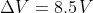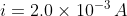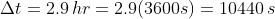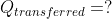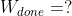Part A) - SolutionThe electric current is given by the formula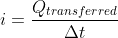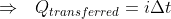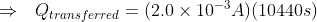or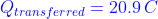Part B) - SolutionThe work done on the charges that passed through the battery can be expressed as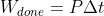where P is the average power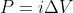thus,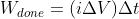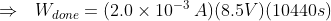or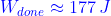#### Earn Coins

Coins can be redeemed for fabulous gifts.

Similar Homework Help Questions
• ### A 6.5 V battery supplies a 3.0 mA current to a circuit for 2.6 hr ....

A 6.5 V battery supplies a 3.0 mA current to a circuit for 2.6 hr . a.How much charge has been transferred from the negative to the positive terminal? answer in unit C b. How much work has been done on the charges that passed through the battery? answer in unit J

• ### A 9.5 V battery supplies a 3.5 mA current to a circuit for 7.0 h ....

A 9.5 V battery supplies a 3.5 mA current to a circuit for 7.0 h . Part A: How much charge has been transferred from the negative to the positive terminal? Part B: How much electric potential energy has been gained by the charges that passed through the battery?

• ### ​A 9V battery supplies a 1.1mA current to a circuit for 5h.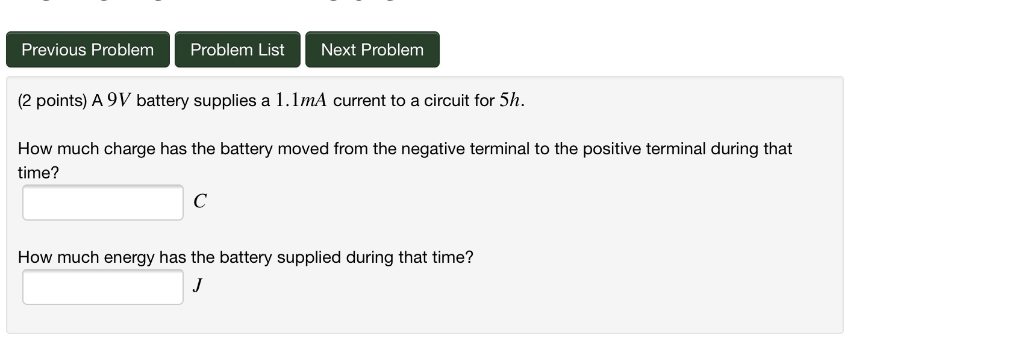A 9V battery supplies a 1.1mA current to a circuit for 5h. How much charge has the battery moved from the negative terminal to the positive terminal during that time? How much energy has the battery supplied during that time?

• ### V(V) A circuit with only one battery, but multiple resistors, has a current of 2.0 A...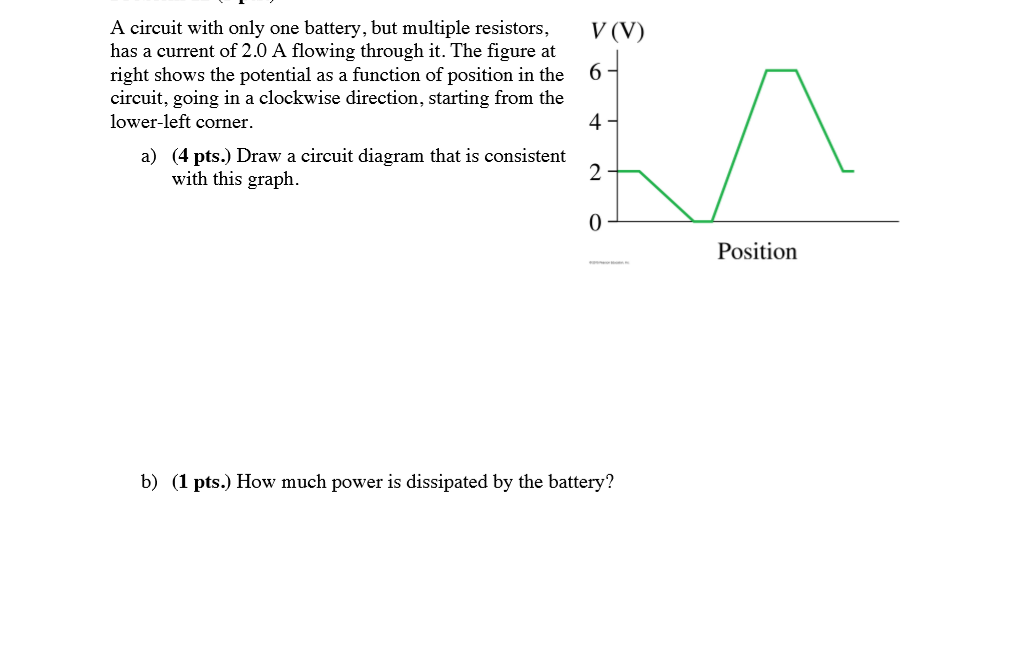V(V) A circuit with only one battery, but multiple resistors, has a current of 2.0 A flowing through it. The figure at right shows the potential as a function of position in the circuit, going in a clockwise direction, starting from the lower-left corner. a) (4 pts.) Draw a circuit diagram that is consistent with this graph. Position b) (1 pts.) How much power is dissipated by the battery?

• ### To recharge a 15.0 V battery, a battery charger must move 4.04×105 C of charge from...

To recharge a 15.0 V battery, a battery charger must move 4.04×105 C of charge from the negative terminal to the positive terminal. How much work is done by the battery charger?

• ### To recharge a 18.0 V battery, a battery charger must move 2.56x105 C of charge from...To recharge a 18.0 V battery, a battery charger must move 2.56x105 C of charge from the negative terminal to the positive terminal How much work is done by the battery charger? Submit Answer Tries 0/12

• ### To recharge a 10 V battery, a battery charger must move 3.7 105 C of charge...

To recharge a 10 V battery, a battery charger must move 3.7 105 C of charge from the negative terminal to the positive terminal. How much work is done by the charger? Express your answer in joules.

• ### 001 10.0 points To recharge a 12 V battery, a battery charger must move 3.8 x...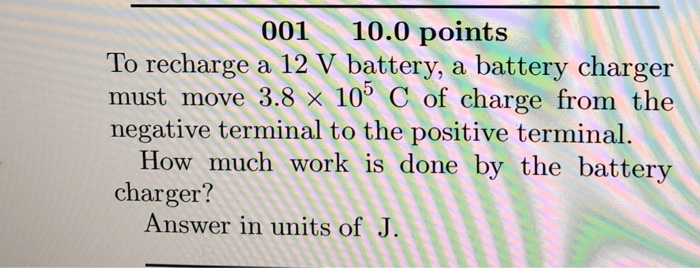001 10.0 points To recharge a 12 V battery, a battery charger must move 3.8 x 105 C of charge from the negative terminal to the positive terminal How much work is done by the battery charger? Answer in units of J

• ### To recharge a 18.0 V battery, a battery charger must move 3.77×105 C of charge from...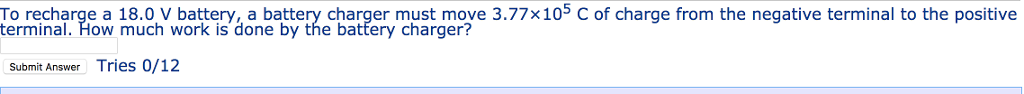To recharge a 18.0 V battery, a battery charger must move 3.77×105 C of charge from the negative terminal to the positive terminal. How much work is done by the batfery charger? Submit Answer Tries 0/12

• ### battery with E = 8.00 V and no internal resistance supplies current to the circuit shown...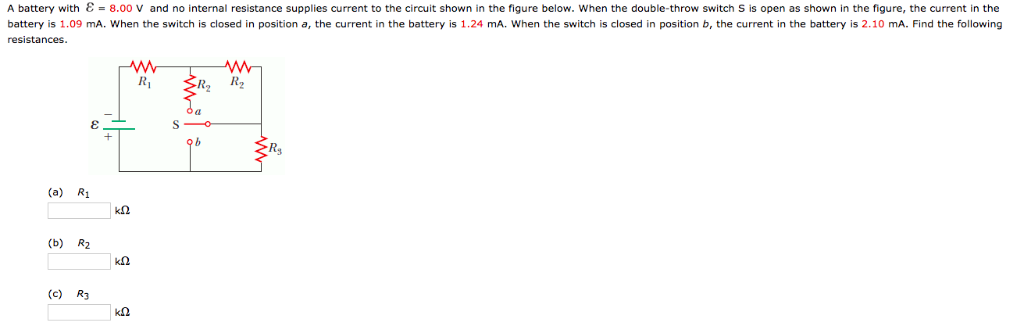battery with E = 8.00 V and no internal resistance supplies current to the circuit shown in the figure below. When the double-throw switch S is open as shown in the figure, the current in the battery is 1.09 mA. When the switch closed in position a, the current the battery is 1.24 mA. When the switch is closed in position b, the current in the battery is 2.10 mA. Find the following resistances. ŠR, Ry (a) R1 k0 (b)...